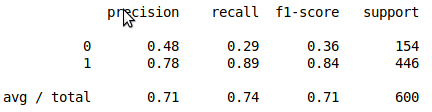# Bagging Classification with Python

Bootstrap aggregation aka bagging is a technique used in machine learning that relies on resampling from the sample and running multiple models from the different samples. The mean or some other value is calculated from the results of each model. For example, if you are using Decisions trees, bagging would have you run the model several times with several different subsamples to help deal with variance in statistics.Bagging is an excellent tool for algorithms that are considered weaker or more susceptible to variances such as decision trees or KNN. In this post, we will use bagging to develop a model that determines whether or not people voted using the turnout dataset. These results will then be compared to a model that was developed in a traditional way.

We will use the turnout dataset available in the pydataset module. Below is some initial code.

```from pydataset import data
import numpy as np
import pandas as pd
from sklearn.model_selection import train_test_split
from sklearn.ensemble import BaggingClassifier
from sklearn.neighbors import KNeighborsClassifier
from sklearn.model_selection import cross_val_score
from sklearn.metrics import classification_report```

We will load our dataset. Then we will separate the independnet and dependent variables from each other and create our train and test sets. The code is below.

```df=data("turnout")
X=df[['age','educate','income',]]
y=df['vote']
X_train,X_test,y_train,y_test=train_test_split(X,y,test_size=.3,random_state=0)```

We can now prepare to run our model. We need to first set up the bagging function. There are several arguments that need to be set. The max_samples argument determines the largest amount of the dataset to use in resampling. The max_features argument is the max number of features to use in a sample. Lastly, the n_estimators is for determining the number of subsamples to draw. The code is as follows

` h=BaggingClassifier(KNeighborsClassifier(n_neighbors=7),max_samples=0.7,max_features=0.7,n_estimators=1000)`

Basically, what we told python was to use up to 70% of the samples, 70% of the features, and make 100 different KNN models that use seven neighbors to classify. Now we run the model with the fit function, make a prediction with the predict function, and check the accuracy with the classificarion_reoirts function.

```h.fit(X_train,y_train)
y_pred=h.predict(X_test)
print(classification_report(y_test,y_pred))```This looks oka below are the results when you do a traditional model without bagging

```X_train,X_test,y_train,y_test=train_test_split(X,y,test_size=.3,random_state=0)
clf=KNeighborsClassifier(7)
clf.fit(X_train,y_train)
y_pred=clf.predict(X_test)
print(classification_report(y_test,y_pred))```The improvement is not much. However, this depends on the purpose and scale of your project. A small improvement can mean millions in the reight context such as for large companies such as Google who deal with billions of people per day.

Conclusion

This post provides an example of the use of bagging in the context of classification. Bagging provides a why to improve your model through the use of resampling.